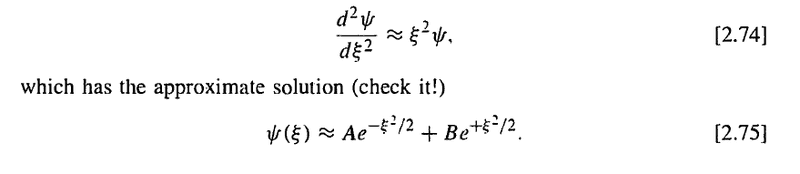# Explanation from Part of Griffith's text (Differential Equation)

tylerscott
This is from section 2.3 in Griffith's book on the harmonic oscillator, and apparently this differential equation should be obvious (to move on in my reading, I need to understand this first). I'm not quite sure how to solve a second order ODE without constant coefficients, so help to get to the given solution of the equation would be more than welcome.
Here is a grab of it:## Answers and Replies

Chopin
Oftentimes, the best way to solve these sorts of equations is just by the time-honored technique of taking an informed guess (a procedure which you justify by giving it the official-sounding name of "ansatz"), and then checking whether it works. In this case, it's fairly straightforward to check that

$$\frac{d^2}{d\xi^2} (A e^{-\xi^2/2} + B e^{\xi^2/2}) = A(\xi^2-1)e^{-\xi^2/2} + B(\xi^2+1)e^{\xi^2/2}$$

Which is an approximate solution to the equation as long as $\xi$ is large.

P.S. I wouldn't worry too much about this approximate solution for anything past a general characterization of the solutions. You're almost certainly going to turn around in the very next section and redo the whole problem using the ladder operator formalism, which allows you to convert the problem into a much simpler first-order equation that you can solve exactly.

Last edited:
Mentor
Actually, Griffiths solves the harmonic oscillator using the algebraic method (ladder operators) first, before describing the analytic method that is being discussed here. Unless he's switched them around for the second edition; I have only the first edition at hand.

tylerscott
Actually, Griffiths solves the harmonic oscillator using the algebraic method (ladder operators) first, before describing the analytic method that is being discussed here. Unless he's switched them around for the second edition; I have only the first edition at hand.

Yeah, you're correct. The ladder operator formalism makes more sense to me than the analytic method.

Chopin
Ah, my bad. I've actually never read Griffiths (blasphemy, I know!) Most of the texts I've seen have started with the analytic method, slogged through it for a while, and then gone "man, that was hard. Hey, let's check out these nifty ladder operators instead--isn't that nicer?"

In any case, since you're only calculating an approximation here, it's difficult to provide any hard and fast rules about how to come up with solutions to the equation. Intuitively, though, you know that the harmonic oscillator is a potential well, so you know the solution is going to be some sort of peaked waveform. The simplest peaked waveform which still tends towards 0 at infinity (so that it's normalizable) is the Gaussian, so something with that form is a logical place to start. Then you just plug it in, and see if it works.

tylerscott
Ok, that makes sense. Thanks